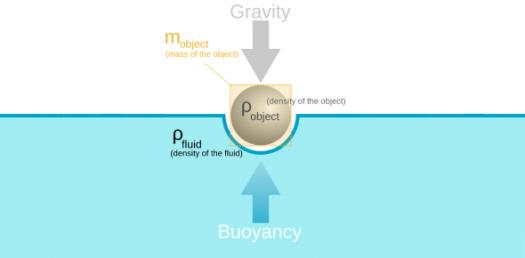# Quiz: Can You Answer The Questions From Density And Buoyancy?

14 Questions | Total Attempts: 1882Settings.

• 1.
Based on the diagram below, rank the three objects from least dense to most dense.
• A.

Object X, object Z, object Y.

• B.

Object Z, object Y, object X.

• C.

Object X, object Y, object Z.

• D.

Object Y, object Z, object X.

• 2.
Fill in the blank: An object is most likely to sink in water if _________________.
• A.

It has a large mass

• B.

It has a large volume

• C.

It has a high density

• D.

It has a low density

• 3.
A ball has a mass of 33.6 grams and a volume of 14.0 cubic centimeters (cc). What is its density?
• A.

470.4 g/cc

• B.

0.42 g/cc

• C.

2.4 g/cc

• D.

19.6 g/cc

• 4.
Three balls were measured and placed in a liquid. Based on the following data, what could be the density of the liquid?
• A.

0.8 g/mL

• B.

0.9 g/mL

• C.

1.0 g/mL

• D.

1.1 g/mL

• 5.
"That's just the tip of the iceberg" is a popular expression you may have heard. It means that what you can see is only a small part of the overall problem. As the diagram shows, most of an iceberg is actually out of sight, below the water level. Based on this diagram, what is the most likely density of the iceberg? (Assume a density of 1.03 g/mL for seawater.)
• A.

0.61 g/cc

• B.

0.88 g/cc

• C.

1.23 g/cc

• D.

0.23 g/cc

• 6.
An object with a density of 0.85 g/cm3 is dropped into each of the two beakers shown below. What will happen to the object in each case?
• A.

It will float in both beakers.

• B.

It will sink in Beaker 1 and float in Beaker 2.

• C.

It will float in Beaker 1 and sink in Beaker 2.

• D.

It will sink in both beakers.

• 7.
Objects 1 and 2 float as shown in the beaker below. The density of the liquid in the beaker is 3.2 g/mL. Which of the densities given below are possible values for the densities of the two objects?
• A.

Density of object 1 = 2.5 g/cm3 Density of object 2 = 3.8 g/cm3

• B.

Density of object 1 = 1.6 g/cm3 Density of object 2 = 2.9 g/cm3

• C.

Density of object 1 = 3.0 g/cm3 Density of object 2 = 1.8 g/cm3

• D.

Density of object 1 = 4.4 g/cm3 Density of object 2 = 4.0 g/cm3

• 8.
Identical objects are placed in Beaker A and in Beaker B. The objects float as shown in the diagrams below. What can you conclude about the liquid in each of the beakers?
• A.

The liquid in Beaker B has a greater density than the liquid in Beaker A.

• B.

The liquid in Beaker A has a greater density than the objects. The liquid in Beaker B is less dense than the objects.

• C.

The liquid in Beaker A has a greater density than the liquid in Beaker B.

• D.

Both liquids are less dense than the objects.

• 9.
Objects 1 and 2 are placed in the beaker below. The density of the liquid in the beaker is 2.0 g/mL. Which of the densities given below are possible values for the densities of the two objects?
• A.

Density of object 1 = 1.5 g/cm3 Density of object 2 = 0.8 g/cm3

• B.

Density of object 1 = 3.8 g/cm3 Density of object 2 = 3.0 g/cm3

• C.

Density of object 1 = 3.0 g/cm3 Density of object 2 = 0.8 g/cm3

• D.

Density of object 1 = 0.8 g/cm3 Density of object 2 = 3.0 g/cm3

• 10.
An object with unknown density is tested in two beakers. Beaker 1 contains liquid with a density of 1.5 g/mL, and Beaker 2 contains liquid with a density of 2.0 g/mL. The object sinks in both beakers. Which of the following statements about the object's density is true?
• A.

The object's density is greater than 2.0 g/cm3.

• B.

The object's density is less than 1.5 g/cm3.

• C.

The object's density is exactly 2.0 g/cm3.

• D.

The object's density is greater than 1.5 g/cm3 and less than 2.0 g/cm3.

• 11.
A rock has a mass of 60g in air.  When it is dipped in water, the mass shown is 40g.  What is the mass of the water that is being displaced by the rock?
• A.

60g

• B.

40g

• C.

20g

• D.

100g

• 12.
A rock has a weight of 4N in the air.  When it is dipped in water, it has a weight of 1N.  What is the buoyant force of the water?
• A.

3N

• B.

4N

• C.

1N

• D.

7N

• 13.
A rock has a mass of 120g in the air, but a mass of only 80g in the water.  How much did the water level go up in the container?
• A.

120mL

• B.

40mL

• C.

80mL

• D.

200mL

• 14.
Which of the following is TRUE?
• A.

A floating object will displace an amount of water equal to the object's volume.

• B.

A sinking object will displace an amount of water equal to the object's mass.

• C.

A floating object displace an amount of water equal to the object's mass.

• D.

A sinking object will displace an amount of water equal to the object's volume.

• E.

A and C are correct.

• F.

C and D are correct

Related TopicsBack to top